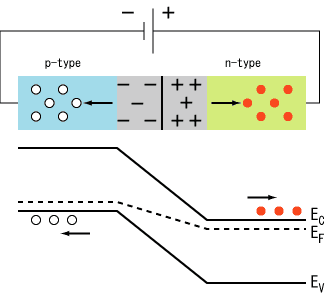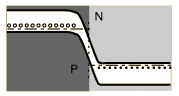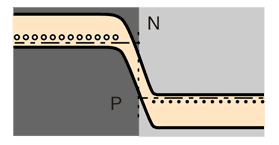# P-n junction diodes (Both bias)

• kev0

#### kev0Given this picture, the circuit is put in reverse bias. Therefore the electrons are flowing INTO the p-type junction.

- As electrons flow into the p-type material the depletion zone is raised as the p-side is made more negative. A picture from hyperphyics.phy-astr.gsu.edu illustrates thisHow does this inhibit current from flowing into the n-junction? Electron current-wise

Conversely the notion of a forward bias diode also confuses me,

- Electrons flow into the n-type , however there still exists that barrier potential from the equilibrium of a p-n junction. How do the electrons 'push through' the barriers to conduct electricity?

Is it because the initial energy from the power source is much greater than the potential barrier?

Thanks,
Kev

Last edited:

This is an old thread that never got answered. I'll do my best to give some pointers as best I can anyway.

For reference, here is an updated link to the hyperphysics website containing the relevant figures and such:
http://hyperphysics.phy-astr.gsu.edu/hbase/Solids/pnjun.html

------------------

Firstly, let me discuss some common confusion that students often experience with these sorts of figures.

The horizontal axis indicates spatial separation (i.e., displacement measured along the PN junction). The vertical axis however represents electrical potential (i.e., energy per unit electrical charge).

So when a PN junction is reversed biased, the electrons enter the P material and move toward the N material. So-far-so-good. But when looking at the figure, where the electrons are moving from left-to-right, the student might think, "the electrons are at a higher potential at the left and are moving to a lower potential at the right, so it should be easy for them to move from left to right: like water flowing downhill. What's stopping it?!"The simple answer is: don't forget: electrons are negatively charged. Electric potentials are defined for positive test charges, not negatively charged electrons. For an electron, it takes energy to go from a higher electric potential to a lower electric potential. Electrons don't flow freely in that direction.

Using a waterfall-like analogy (where "up" is higher electrical potential) doesn't really work so well for electrons. Under their own accord, electrons would rather float up; it takes energy to push them down.

------------------------

That's the simple answer. The more realistic answer is that as one increases the external potential across the reverse biased PN junction, the depletion layer widens, increasing the resistance across the region. The potential difference across the PN junction rises to essentially match the externally applied voltage. Little to no current flows.

----------------------

In the case of the forward biased case, the depletion region narrows, lowering the resistance, making it relatively easy for the electrons to flow.In terms of waterfall like analogy (where it takes energy to push electrons "down"), in forward bias it's quite easy to push them down enough such that easily and freely flow through the PN junction when the applied voltage is greater than the PN junction's equilibrium potential.

•berkeman and Greg Bernhardt